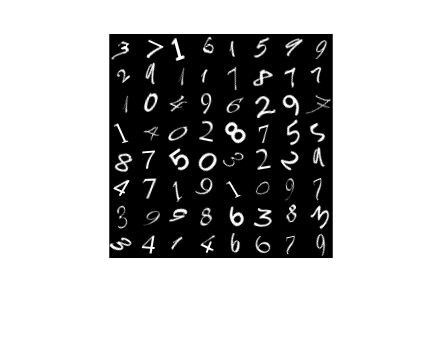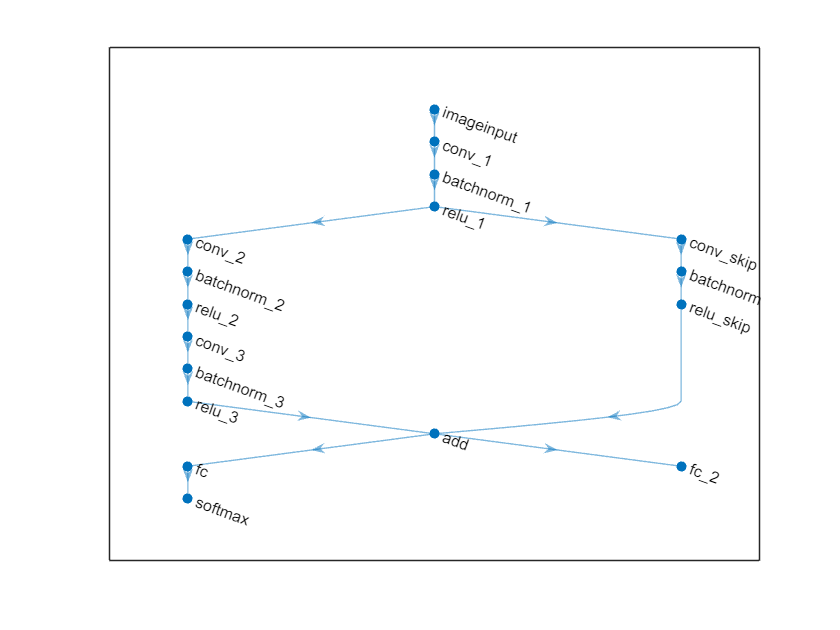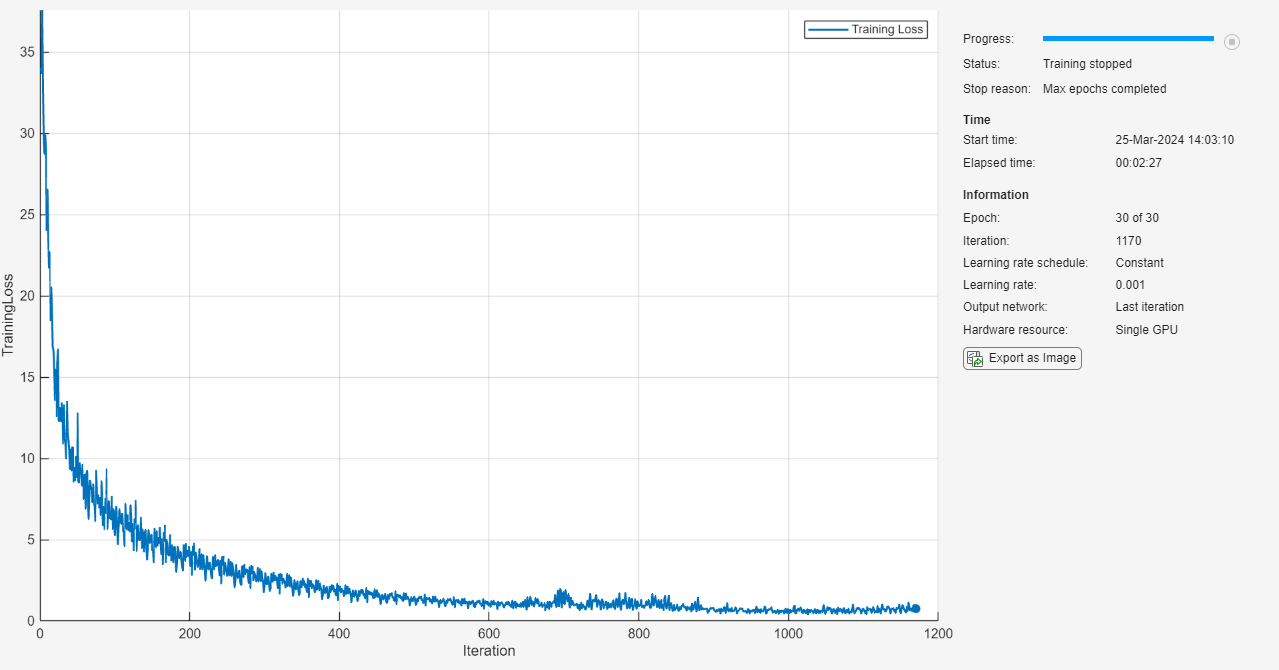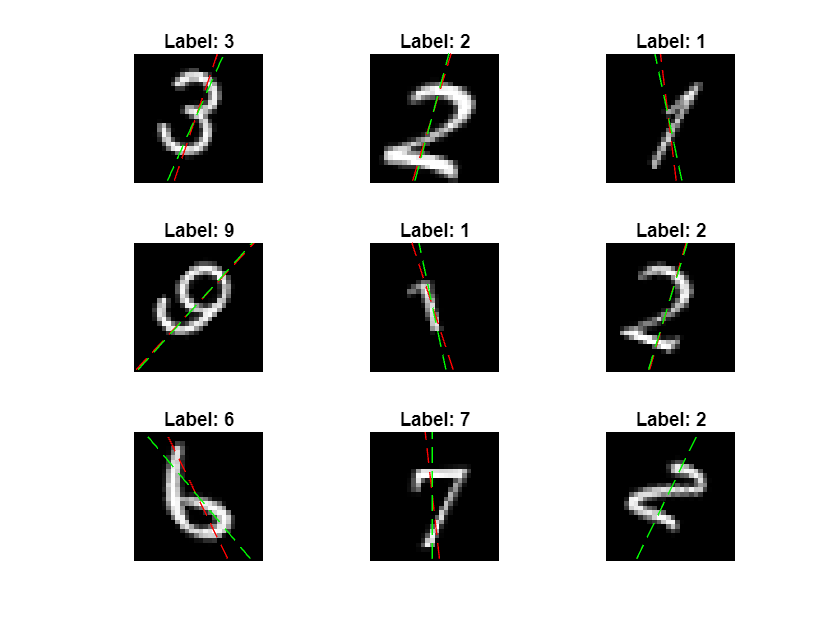Main Content

# Train Network with Multiple Outputs

This example shows how to train a deep learning network with multiple outputs that predict both labels and angles of rotations of handwritten digits.

To train a network with multiple outputs, you must train the network using a custom training loop.

### Load Training Data

The `digitTrain4DArrayData` function loads the images, their digit labels, and their angles of rotation from the vertical. Create an `arrayDatastore` object for the images, labels, and the angles, and then use the `combine` function to make a single datastore that contains all of the training data. Extract the class names and number of nondiscrete responses.

```[XTrain,YTrain,anglesTrain] = digitTrain4DArrayData; dsXTrain = arrayDatastore(XTrain,'IterationDimension',4); dsYTrain = arrayDatastore(YTrain); dsAnglesTrain = arrayDatastore(anglesTrain); dsTrain = combine(dsXTrain,dsYTrain,dsAnglesTrain); classNames = categories(YTrain); numClasses = numel(classNames); numObservations = numel(YTrain);```

View some images from the training data.

```idx = randperm(numObservations,64); I = imtile(XTrain(:,:,:,idx)); figure imshow(I)```### Define Deep Learning Model

Define the following network that predicts both labels and angles of rotation.

• A convolution-batchnorm-ReLU block with 16 5-by-5 filters.

• Two convolution-batchnorm-ReLU blocks each with 32 3-by-3 filters.

• A skip connection around the previous two blocks containing a convolution-batchnorm-ReLU block with 32 1-by-1 convolutions.

• Merge the skip connection using addition.

• For classification output, a branch with a fully connected operation of size 10 (the number of classes) and a softmax operation.

• For the regression output, a branch with a fully connected operation of size 1 (the number of responses).

Define the main block of layers as a layer graph.

```layers = [ imageInputLayer([28 28 1],'Normalization','none','Name','in') convolution2dLayer(5,16,'Padding','same','Name','conv1') batchNormalizationLayer('Name','bn1') reluLayer('Name','relu1') convolution2dLayer(3,32,'Padding','same','Stride',2,'Name','conv2') batchNormalizationLayer('Name','bn2') reluLayer('Name','relu2') convolution2dLayer(3,32,'Padding','same','Name','conv3') batchNormalizationLayer('Name','bn3') reluLayer('Name','relu4') additionLayer(2,'Name','addition') fullyConnectedLayer(numClasses,'Name','fc1') softmaxLayer('Name','softmax')]; lgraph = layerGraph(layers);```

Add the skip connection.

```layers = [ convolution2dLayer(1,32,'Stride',2,'Name','convSkip') batchNormalizationLayer('Name','bnSkip') reluLayer('Name','reluSkip')]; lgraph = addLayers(lgraph,layers); lgraph = connectLayers(lgraph,'relu1','convSkip'); lgraph = connectLayers(lgraph,'reluSkip','addition/in2');```

Add the fully connected layer for regression.

```layers = fullyConnectedLayer(1,'Name','fc2'); lgraph = addLayers(lgraph,layers); lgraph = connectLayers(lgraph,'addition','fc2');```

View the layer graph in a plot.

```figure plot(lgraph)```Create a `dlnetwork` object from the layer graph.

`dlnet = dlnetwork(lgraph)`
```dlnet = dlnetwork with properties: Layers: [17×1 nnet.cnn.layer.Layer] Connections: [17×2 table] Learnables: [20×3 table] State: [8×3 table] InputNames: {'in'} OutputNames: {'softmax' 'fc2'} ```

#### Define Model Gradients Function

Create the function `modelGradients`, listed at the end of the example, that takes as input, the `dlnetwork` object `dlnet`, a mini-batch of input data `dlX` with corresponding targets `T1` and `T2` containing the labels and angles, respectively, and returns the gradients of the loss with respect to the learnable parameters, the updated network state, and the corresponding loss.

### Specify Training Options

Specify the training options. Train for 30 epochs using a mini-batch size of 128.

```numEpochs = 30; miniBatchSize = 128;```

Visualize the training progress in a plot.

`plots = "training-progress";`

### Train Model

Use `minibatchqueue` to process and manage the mini-batches of images. For each mini-batch:

• Use the custom mini-batch preprocessing function `preprocessMiniBatch` (defined at the end of this example) to one-hot encode the class labels.

• Format the image data with the dimension labels `'SSCB'` (spatial, spatial, channel, batch). By default, the `minibatchqueue` object converts the data to `dlarray` objects with underlying type `single`. Do not add a format to the class labels or angles.

• Train on a GPU if one is available. By default, the `minibatchqueue` object converts each output to a `gpuArray` if a GPU is available. Using a GPU requires Parallel Computing Toolbox™ and a supported GPU device. For information on supported devices, see GPU Support by Release (Parallel Computing Toolbox).

```mbq = minibatchqueue(dsTrain,... 'MiniBatchSize',miniBatchSize,... 'MiniBatchFcn', @preprocessData,... 'MiniBatchFormat',{'SSCB','',''});```

Train the model using a custom training loop. For each epoch, shuffle the data and loop over mini-batches of data. At the end of each iteration, display the training progress. For each mini-batch:

• Evaluate the model gradients and loss using `dlfeval` and the `modelGradients` function.

• Update the network parameters using the `adamupdate` function.

Initialize the training progress plot.

```if plots == "training-progress" figure lineLossTrain = animatedline('Color',[0.85 0.325 0.098]); ylim([0 inf]) xlabel("Iteration") ylabel("Loss") grid on end```

Initialize parameters for Adam.

```trailingAvg = []; trailingAvgSq = [];```

Train the model.

```iteration = 0; start = tic; % Loop over epochs. for epoch = 1:numEpochs % Shuffle data. shuffle(mbq) % Loop over mini-batches while hasdata(mbq) iteration = iteration + 1; [dlX,dlY1,dlY2] = next(mbq); % Evaluate the model gradients, state, and loss using dlfeval and the % modelGradients function. [gradients,state,loss] = dlfeval(@modelGradients, dlnet, dlX, dlY1, dlY2); dlnet.State = state; % Update the network parameters using the Adam optimizer. [dlnet,trailingAvg,trailingAvgSq] = adamupdate(dlnet,gradients, ... trailingAvg,trailingAvgSq,iteration); % Display the training progress. if plots == "training-progress" D = duration(0,0,toc(start),'Format','hh:mm:ss'); addpoints(lineLossTrain,iteration,double(gather(extractdata(loss)))) title("Epoch: " + epoch + ", Elapsed: " + string(D)) drawnow end end end```### Test Model

Test the classification accuracy of the model by comparing the predictions on a test set with the true labels and angles. Manage the test data set using a `minibatchqueue` object with the same setting as the training data.

```[XTest,Y1Test,anglesTest] = digitTest4DArrayData; dsXTest = arrayDatastore(XTest,'IterationDimension',4); dsYTest = arrayDatastore(Y1Test); dsAnglesTest = arrayDatastore(anglesTest); dsTest = combine(dsXTest,dsYTest,dsAnglesTest); mbqTest = minibatchqueue(dsTest,... 'MiniBatchSize',miniBatchSize,... 'MiniBatchFcn', @preprocessData,... 'MiniBatchFormat',{'SSCB','',''});```

To predict the labels and angles of the validation data, loop over the mini-batches and use the `predict` function. Store the predicted classes and angles. Compare the predicted and true classes and angles and store the results.

```classesPredictions = []; anglesPredictions = []; classCorr = []; angleDiff = []; % Loop over mini-batches. while hasdata(mbqTest) % Read mini-batch of data. [dlXTest,dlY1Test,dlY2Test] = next(mbqTest); % Make predictions using the predict function. [dlY1Pred,dlY2Pred] = predict(dlnet,dlXTest,'Outputs',["softmax" "fc2"]); % Determine predicted classes. Y1PredBatch = onehotdecode(dlY1Pred,classNames,1); classesPredictions = [classesPredictions Y1PredBatch]; % Dermine predicted angles Y2PredBatch = extractdata(dlY2Pred); anglesPredictions = [anglesPredictions Y2PredBatch]; % Compare predicted and true classes Y1Test = onehotdecode(dlY1Test,classNames,1); classCorr = [classCorr Y1PredBatch == Y1Test]; % Compare predicted and true angles angleDiffBatch = Y2PredBatch - dlY2Test; angleDiff = [angleDiff extractdata(gather(angleDiffBatch))]; end ```

Evaluate the classification accuracy.

`accuracy = mean(classCorr)`
```accuracy = 0.9814 ```

Evaluate the regression accuracy.

`angleRMSE = sqrt(mean(angleDiff.^2))`
```angleRMSE = single 7.7431 ```

View some of the images with their predictions. Display the predicted angles in red and the correct labels in green.

```idx = randperm(size(XTest,4),9); figure for i = 1:9 subplot(3,3,i) I = XTest(:,:,:,idx(i)); imshow(I) hold on sz = size(I,1); offset = sz/2; thetaPred = anglesPredictions(idx(i)); plot(offset*[1-tand(thetaPred) 1+tand(thetaPred)],[sz 0],'r--') thetaValidation = anglesTest(idx(i)); plot(offset*[1-tand(thetaValidation) 1+tand(thetaValidation)],[sz 0],'g--') hold off label = string(classesPredictions(idx(i))); title("Label: " + label) end```### Model Gradients Function

The `modelGradients` function, takes as input, the `dlnetwork` object `dlnet`, a mini-batch of input data `dlX` with corresponding targets `T1` and `T2` containing the labels and angles, respectively, and returns the gradients of the loss with respect to the learnable parameters, the updated network state, and the corresponding loss.

```function [gradients,state,loss] = modelGradients(dlnet,dlX,T1,T2) [dlY1,dlY2,state] = forward(dlnet,dlX,'Outputs',["softmax" "fc2"]); lossLabels = crossentropy(dlY1,T1); lossAngles = mse(dlY2,T2); loss = lossLabels + 0.1*lossAngles; gradients = dlgradient(loss,dlnet.Learnables); end```

### Mini-Batch Preprocessing Function

The `preprocessMiniBatch` function preprocesses the data using the following steps:

1. Extract the image data from the incoming cell array and concatenate into a numeric array. Concatenating the image data over the fourth dimension adds a third dimension to each image, to be used as a singleton channel dimension.

2. Extract the label and angle data from the incoming cell arrays and concatenate along the second dimension into a categorical array and a numeric array, respectively.

3. One-hot encode the categorical labels into numeric arrays. Encoding into the first dimension produces an encoded array that matches the shape of the network output.

```function [X,Y,angle] = preprocessData(XCell,YCell,angleCell) % Extract image data from cell and concatenate X = cat(4,XCell{:}); % Extract label data from cell and concatenate Y = cat(2,YCell{:}); % Extract angle data from cell and concatenate angle = cat(2,angleCell{:}); % One-hot encode labels Y = onehotencode(Y,1); end```

Download ebook